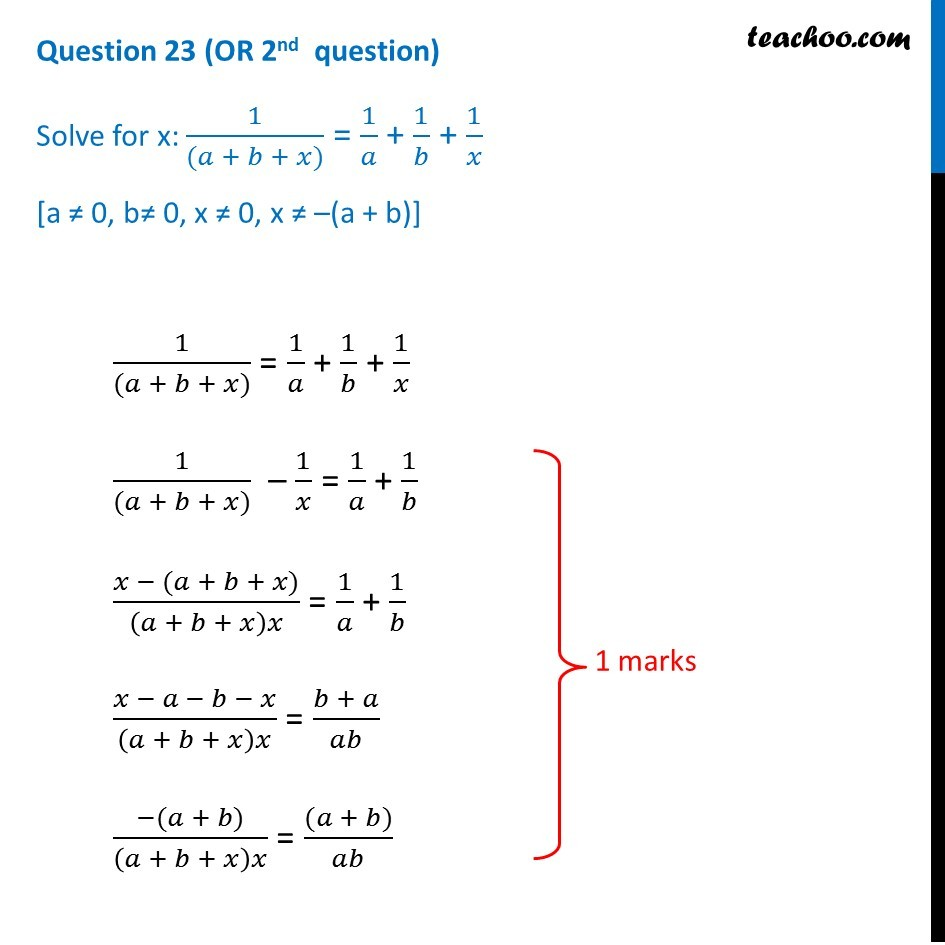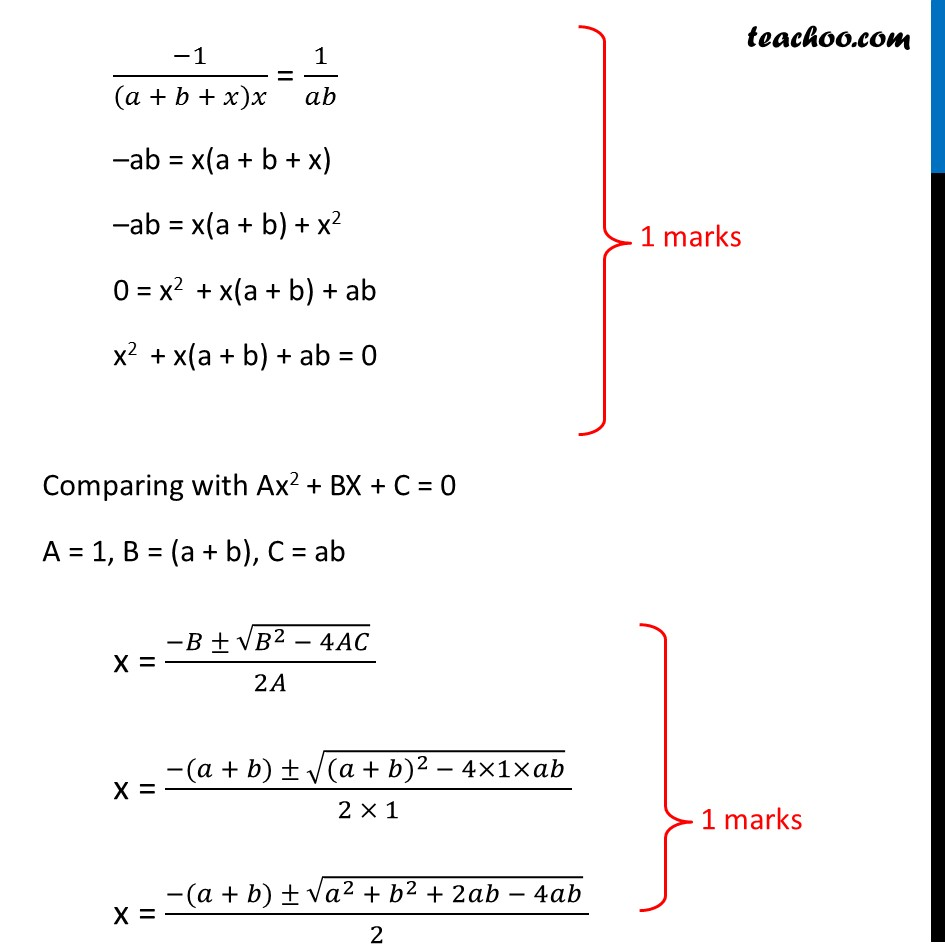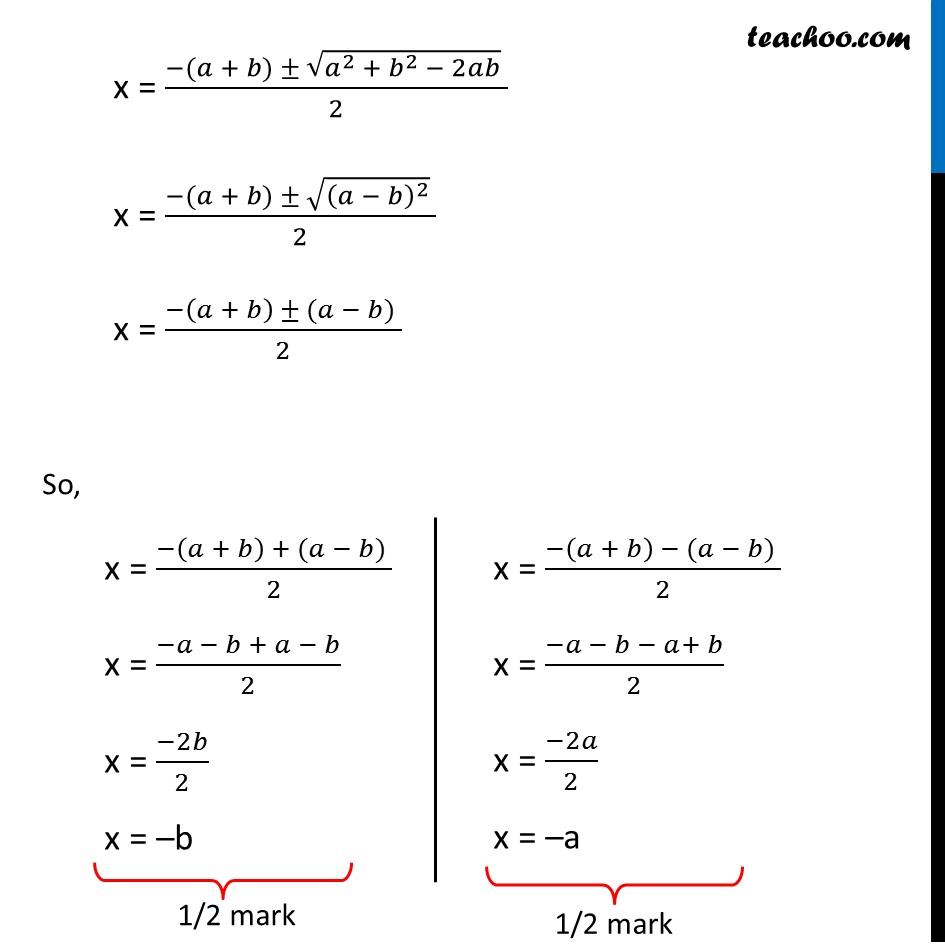Question 23 (OR 2 nd   question)

Solve for x: 1/((a + b + x)) = 1/a + 1/b + 1/x

[a ≠ 0, b ≠ 0, x ≠ 0, x ≠ –(a + b)]Subscribe to our Youtube Channel - https://you.tube/teachoo

1. Class 10
2. Solutions of Sample Papers for Class 10 Boards
3. CBSE Class 10 Sample Paper for 2019 Boards

Transcript

Question 23 (OR 2nd question) Solve for x: 1/((𝑎 + 𝑏 + 𝑥)) = 1/𝑎 + 1/𝑏 + 1/𝑥 [a ≠ 0, b ≠ 0, x ≠ 0, x ≠ –(a + b)] 1/((𝑎 + 𝑏 + 𝑥)) = 1/𝑎 + 1/𝑏 + 1/𝑥 1/((𝑎 + 𝑏 + 𝑥)) – 1/𝑥 = 1/𝑎 + 1/𝑏 (𝑥 − (𝑎 + 𝑏 + 𝑥))/(𝑎 + 𝑏 + 𝑥)𝑥 = 1/𝑎 + 1/𝑏 (𝑥 − 𝑎 − 𝑏 − 𝑥)/(𝑎 + 𝑏 + 𝑥)𝑥 = (𝑏 + 𝑎)/𝑎𝑏 (−(𝑎 + 𝑏))/(𝑎 + 𝑏 + 𝑥)𝑥 = ((𝑎 + 𝑏))/𝑎𝑏 (−1)/(𝑎 + 𝑏 + 𝑥)𝑥 = 1/𝑎𝑏 –ab = x(a + b + x) –ab = x(a + b) + x2 0 = x2 + x(a + b) + ab x2 + x(a + b) + ab = 0 Comparing with Ax2 + BX + C = 0 A = 1, B = (a + b), C = ab x = (−𝐵 ± √(𝐵^(2 )− 4𝐴𝐶) )/2𝐴 x = (−(𝑎 + 𝑏) ± √(〖(𝑎 + 𝑏)〗^(2 )− 4×1×𝑎𝑏) )/(2 × 1) x = (−(𝑎 + 𝑏) ± √(𝑎^2 + 𝑏^2 + 2𝑎𝑏 − 4𝑎𝑏) )/2 x = (−(𝑎 + 𝑏) ± √(𝑎^2 + 𝑏^2 − 2𝑎𝑏) )/2 x = (−(𝑎 + 𝑏) ± √((𝑎 − 𝑏)^2 ) )/2 x = (−(𝑎 + 𝑏) ± (𝑎 − 𝑏) )/2 So, x = (−(𝑎 + 𝑏) + (𝑎 − 𝑏) )/2 x = (−𝑎 − 𝑏 + 𝑎 − 𝑏)/2 x = (−2𝑏)/2 x = –b x = (−(𝑎 + 𝑏) − (𝑎 − 𝑏) )/2 x = (−𝑎 − 𝑏 − 𝑎+ 𝑏)/2 x = (−2𝑎)/2 x = –a

CBSE Class 10 Sample Paper for 2019 Boards

Class 10
Solutions of Sample Papers for Class 10 Boards